﻿ 基于近似动态规划的目标追踪控制算法<sup>*</sup>Download PDF文章快速检索 高级检索

1. 北京航空航天大学 宇航学院, 北京 100083;
2. 北京航天自动控制研究所, 北京 100854

Target tracking control algorithm based on approximate dynamic programming
LI Huifeng1, YI Wenfeng1, CHENG Xiaoming1,2
1. School of Astronautics, Beihang University, Beijing 100083, China;
2. Beijing Aerospace Automatic Control Institute, Beijing 100854, China
Received: 2018-06-12; Accepted: 2018-09-14; Published online: 2018-11-20 09:32
Corresponding author. LI Huifeng, E-mail:lihuifeng@buaa.edu.cn
Abstract: The control algorithm for the target tracking problem cannot be well adapted to the problem of large-scale maneuver flight or even game with us. This paper proposes a control algorithm for target tracking using approximate dynamic programming. The game algorithm is used to train our UAV to form an experience. The positions of both sides are taken as known quantity and the roll direction as the control quantity. The relative positions of two objects are used to derive their features and then an approximate function is formed. The rollout algorithm is used to obtain the optimal decision, and the flexible and effective tracking of tracking targets and even gaming targets can be achieved. The simulation results verify the effectiveness of approximate dynamic programming for control algorithms.
Keywords: approximate dynamic programming     target tracking     flight control     optimal decision     game

1 近似动态规划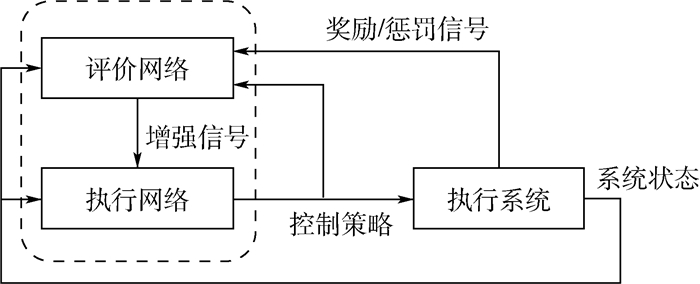图 1 近似动态规划结构 Fig. 1 ADP structure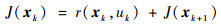(1)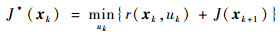(2)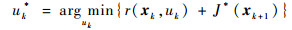(3)

 变量 说明 x 状态矢量 xi 在第i步的状态 xn X的第n个状态矢量 xterm 特殊的终止状态 xpos 无人机x坐标 ypos 无人机y坐标 X 状态矢量[x1, x2, …, xn]T f(x, u) 状态转移函数 π(x) 机动策略 π*(x) 最佳机动策略 π(x) 通过滚动算法生成的策略 J(x) 状态x的未来奖励值 Jk(x) J(x)的第k次迭代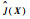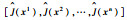Japprox(x) J(x)的函数逼近形式 S(x) 无人机的评估函数 γ 奖励折扣因子 u 控制或移动动作 ζ(x) 状态x的特征向量 β 函数参数向量 g(x) 目标奖励函数 gpa(x) 优势位置函数 pt 终止函数的概率 T Bellman逆操作因子 J*(x) J(x)的最佳值

2 算法构架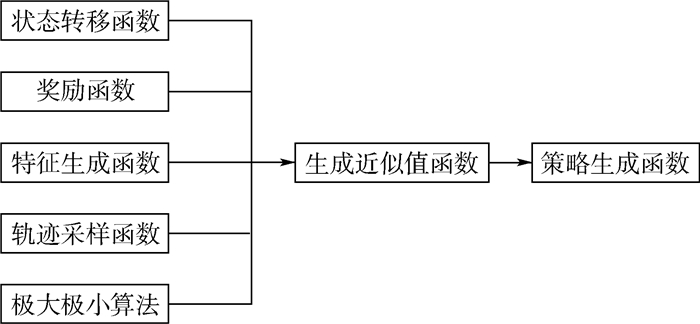图 2 算法框架 Fig. 2 Algorithm framework
2.1 状态量、目标、控制量以及动力学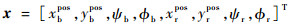(4)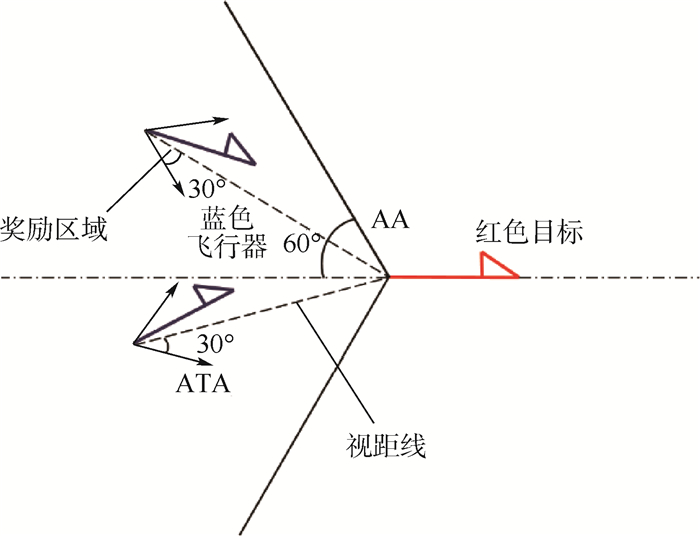图 3 奖励区域 Fig. 3 Reward area
 算法1  优势位置函数gpa(x) 输入:{x}。 R=“飞行器与目标的欧几里得距离” if(0.1 m < R < 3.0 m) & (|AA| < 60°) & (|ATA| < 30°) then gpa(x)=1.0 else gpa(x)=0 end if 输出奖励:(gpa)。

 算法2   状态转移函数f(xi, ub, ur) 输入:{xi, ub, ur}。 for i=1:5(once per Δt=0.05 s) do for{red, blue} do (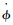=40(°)/s, ϕrmax=18°, ϕbmax=23°) if u=L then ϕ=max(ϕ－Δt, －ϕmax) else if u=R then ϕ=min(ϕ+Δt, ϕmax) end if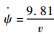tan ϕ(assume v=2.5 m/s) ψ=ψ+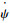Δt; xpos=xpos+Δtvsin ψ ypos=ypos+Δtvcos ψ end for end for

2.2 奖励函数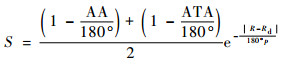(5)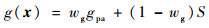(6)